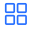Javascript 异步编程前端频道

0

0

``````\$.post("/foo.json", function (dataOfFoo) {//多层套嵌结构的Ajax回调
\$.post("/bar.json", function (dataOfBar) {
\$.post("/baz.json", function (dataOfBaz) {
});
});
});

function throwError(){
throw new Error('ERROR');
}

try{
setTimeout(throwError, 3000);
} catch(e){
}
``````

``````var Queue = function(){
this.list = []
}
Queue.prototype = {
constructor:Queue,
queue:function(fn) {
this.list.push(fn)
return this;
},
dequeue:function(){
var fn = this.list.shift()||function(){};
fn.call(this)
}
}
``````

``````var q = new Queue;
q.queue(function(){
log(1)
}).queue(function(){
log(2)
}).queue(function(){
log(3)
});
while(q.list.length){
q.dequeune();
}
``````

``````var el = document.getElementById("test");
var q = new Queue();
q.queue(function(){
var self = this;
el.innerHTML = 1
setTimeout(function(){
self.dequeue()
},1000);
}).queue(function(){
var self = this;
el.innerHTML = 2
setTimeout(function(){
self.dequeue()
},1000);
}).queue(function(){
var self = this;
el.innerHTML = 3
setTimeout(function(){
self.dequeue()
},1000);
}).dequeue()
``````

``````var Queue = function(){
this.list = []
}
Queue.prototype = {
constructor:Queue,
queue:function(fn) {
this.list.push(fn)
return this;
},
wait:function(ms){
this.list.push(ms)
return this;
},
dequeue:function(){
var self = this, list = self.list;
var el = list.shift()||function(){};
if(typeof el == "number"){
setTimeout(function(){
self.dequeue();
},el);
}else if(typeof el == "function") {
el.call(this)
if(list.length)
self.dequeue();
}
}
}
``````

Great，如果我们能自由控制每个回调的间隔，这对于做动画效果说，就变得非常简单了。但这Queue类相对我们最初定下的目标来说，还是差得远。Ajax，多投事件，domReay将统统划归于它的麾下，因此它需要用一些适用性更强的API。用过dojo的人也知，它的Deferred就像DNA的染色体一样，是双线的，可以捕捉不在同一时间线上的异常，而且这些列队不能像卫生筷那样用完一次就废了，这样就无法支撑多投事件的实现了。想要实现这些功能，就需要一个很复杂的东西，我将在第二部分隆重介绍我的异步列队，看它是如何优雅地解决这些问题。